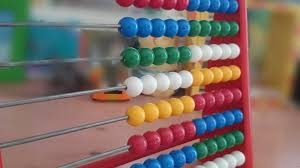MasterIGCSE - Maths# MasterIGCSE - Maths

Liên hệ

CIE, IGCSE Maths (0580) Extended & Core courses

Teacher: Ms Hong, 8 years of teaching Cambridge KS3, IGCSE, Alevel and IB curriculum at BIS and UNIS Hanoi

Khóa học được thiết kế giúp thí sinh có chuẩn bị tốt nhất cho kì thi mùa hè. Học sinh có cơ hội ôn tập toàn bộ chương trình, bộ đề và kĩ năng thi cho đề IGCSE Maths (0580) với gia sư có kinh nghiệm ôn thi nhiều năm chuyên với ban khảo thí của Cambridge.

These courses are designed to prepare the students for the summer exams. Students will have the chance to revise key aspects of the entire Maths (0580) syllabus, practice exam questions and improve their exam technique in separate classes for Cambridge International Examination - CIE exam board, taught by experienced professional tutors.

1. Number (15hours)
• Basic numbers, rounding, estimation
• Set Venn diagrams
• Conversion: Fractions, Decimals, Percentages
• Standard forms
• Bounds
• Ratio and proportions
• Time, currency conversions
• Financial problems
• Exponential growth and decay (compound int.)
1. Algebra (22.5hours)
• Using algebra to solve problems
• Factorization (linear)
• Algebraic fractions
• Index notations
• Linear equations
• Simultaneous linear equations
• Linear inequalities
• Graphical inequalities
• Sequences and nth term
• Direct/ Inverse proportionally
• Kinematics, distance-time and speed-time graph
• Constructing Graphs & Solving Equations Graphically
• Functions

1. Shape and space (15 hours)
• Properties of Shapes
• Construction
• Similarity
• Symmetry (inc. Circles)
• ​Loci
• 2D Shapes: Perimeters & Areas
• Circle Problems (Area, Circumference, Arcs)
• 3D Shapes: Volumes & Surface Areas
• ​Equations of a Line (gradients, mid-points, perpendicular & parallel lines)
•
1. Trigonometry (9hours)
• Bearings
• 2D Pythagoras & Trigonometry (SOHCAHTOA)
• Sine & Cosine Rule
• 3D Pythagoras & Trigonometry

1.  Matrices and transformations (9 hours)
• Vectors
• Transformations (Reflection, Enlargement, Rotation, Translation)
• Matrices

1. Probability and Statistics (12hours)
• Basic probability and relative frequency
• Probability – tree diagrams and combine events
• Basic statistical diagrams and Stem-and-leaf
• Scatter graphs
• Mean, mode, median and range
• Cumulative frequency
• Histograms

Tài liệu chia sẻ

1. Statistics